Homework Help Question & Answers

# For the compound ABC4 where A is hydrogen, B is a halogen and C is a...

For the compound ABC4 where A is hydrogen, B is a halogen and C is a chalcogen,

a) Indicate the total valence electrons

b) Draw a Lewis structure and any associated resonance structures. Other information, B is the least electronegative and can have an expanded octet.

c) From your structure, what is the molecular shape of each central element

d) Is your drawn structure polar or non polar

#### Homework Answers

Answer #1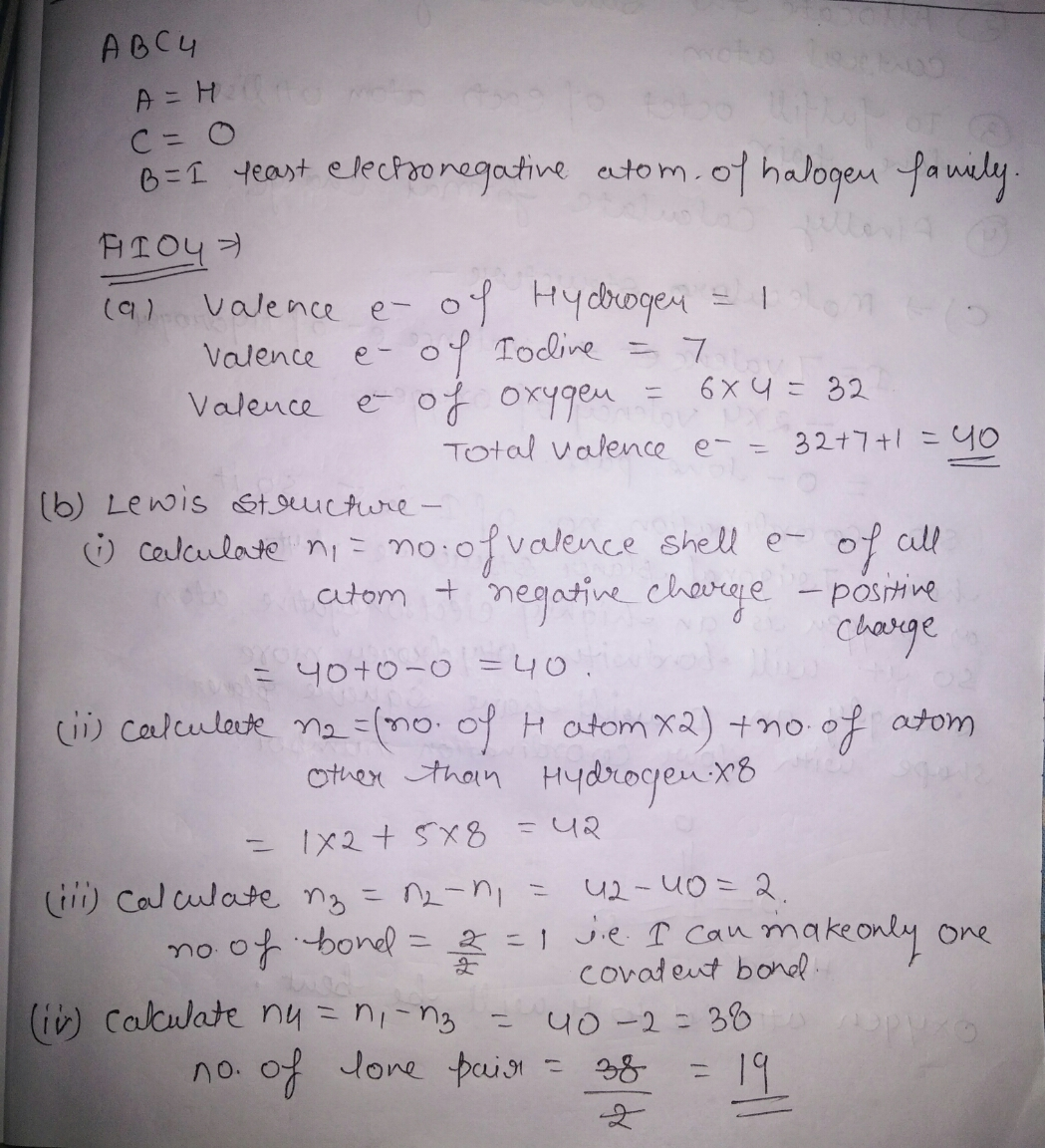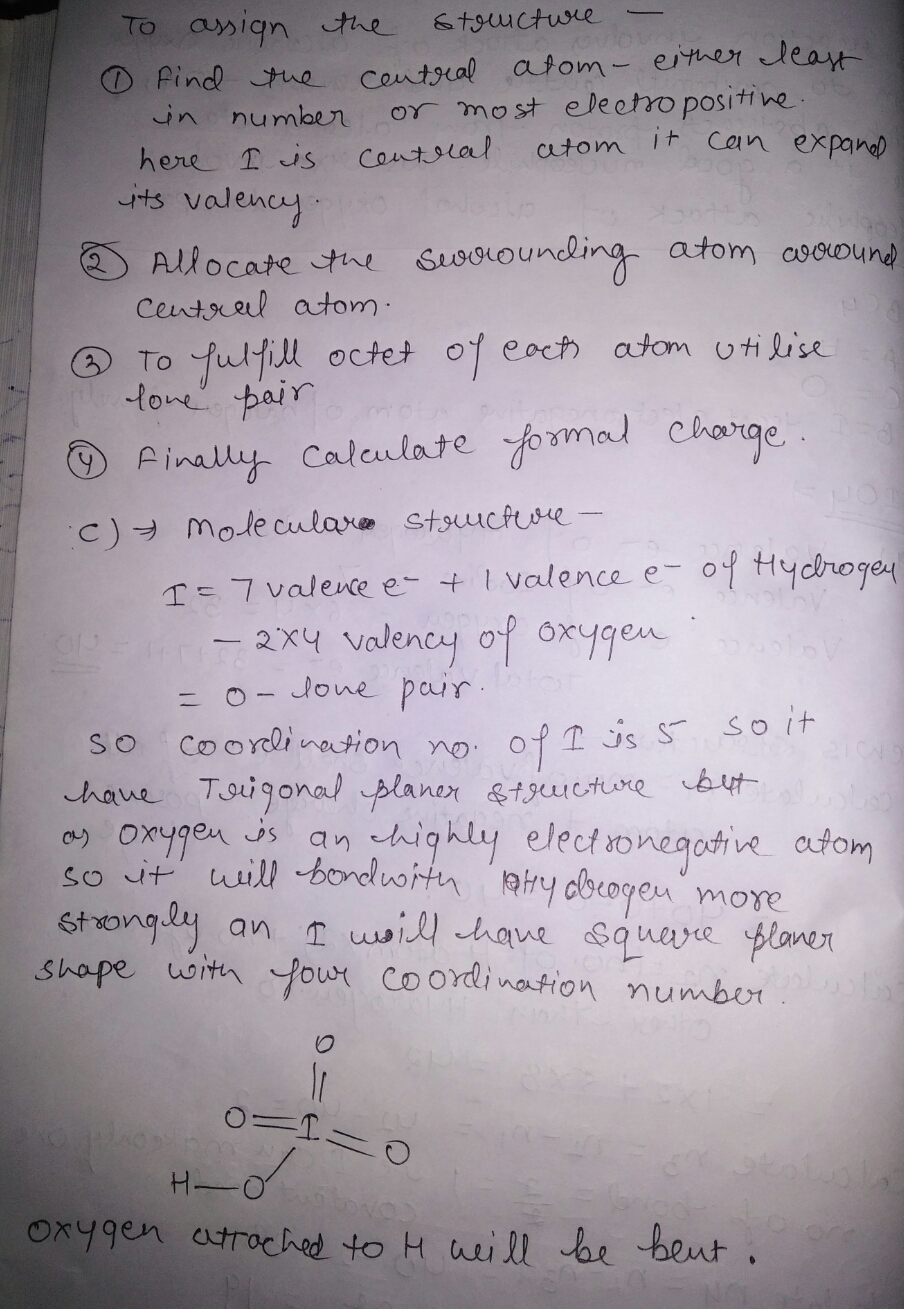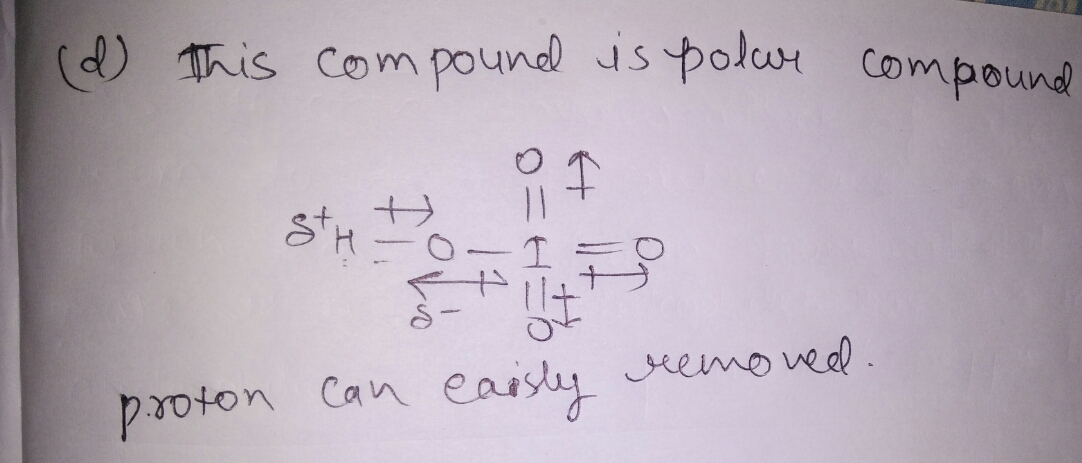Know the answer?
Your Answer:

#### Post as a guest

Your Name:

What's your source?

#### Earn Coin

Coins can be redeemed for fabulous gifts.

Not the answer you're looking for? Ask your own homework help question. Our experts will answer your question WITHIN MINUTES for Free.
Similar Homework Help Questions
• ### A compound designated as AB2C, has a total valence electrons of 26 where each element B...

A compound designated as AB2C, has a total valence electrons of 26 where each element B and C has 7 valence electrons. Element A is the least electronegative. Draw a reasonable Lewis structure. What is the molecular shape of your Lewis structure Is your structure polar or non polar

• ### Balance the following chemical equations AB   + C2    à A8C18   + C2B     ABC3 + A2D   ->  ...

Balance the following chemical equations AB   + C2    à A8C18   + C2B     ABC3 + A2D   ->   A10B10D15   + AC A compound designated as AB2C, has a total valence electrons of 26 where each element B and C has 7 valence electrons. Element A is the least electronegative. Draw a reasonable Lewis structure. What is the molecular shape of your Lewis structure Is your structure polar or non polar A 33 g hot piece of metal at 450K is placed in...

• ### 1. A compound designated as AB,C, has a total valence electron of 26 where each element...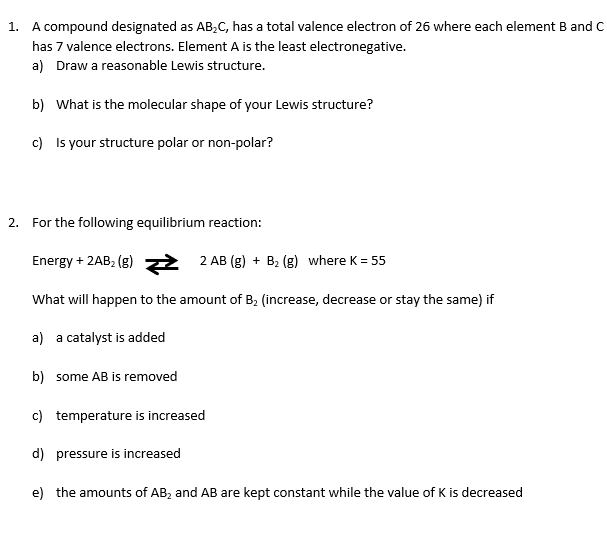1. A compound designated as AB,C, has a total valence electron of 26 where each element B and C has 7 valence electrons. Element A is the least electronegative. a) Draw a reasonable Lewis structure. b) What is the molecular shape of your Lewis structure? c) Is your structure polar or non-polar? 2. For the following equilibrium reaction: Energy + 2AB (g) = 2 AB (g) + B2 (g) where K = 55 What will happen to the amount of...

• ### Experiment 11 Formula Central atom: Lewis Structure: Species Geometry: Element: Center 1 Center 2 Valence electrons...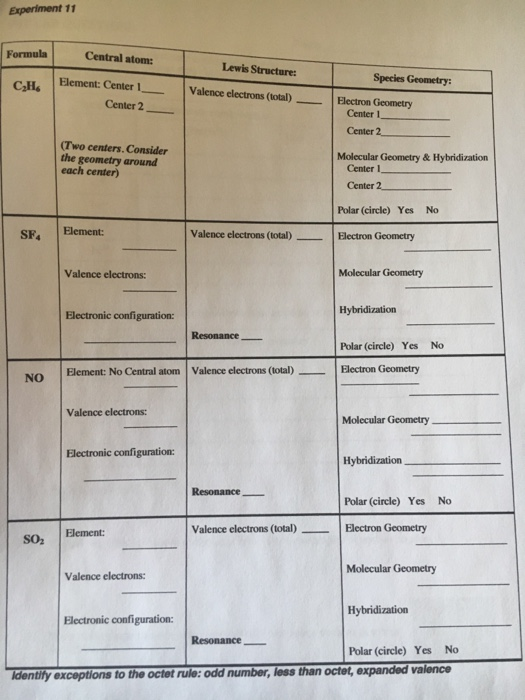Experiment 11 Formula Central atom: Lewis Structure: Species Geometry: Element: Center 1 Center 2 Valence electrons (total) C2Hs Geometry Center Center 2 Two centers Consider the geometry around Molecular Geometry & Hybridization each center) Center Center 2. Polar (circle) Yes No SF. Element: Valence electrons (total) Electron Geometry Molecular Geometry Valence electrons: Hybridization Electronic configuration: Polar (circle) Yes No No Element: No Central atom Valence electrons (total) Electron Geometry Valence electrons: Molecular Geometry Electronic configuration: Hybridization Resonance Polar (circle) Yes...

• ### VSEPR PRACTICE Fill in the table below for these molecules. Please perform your calculations on scratch...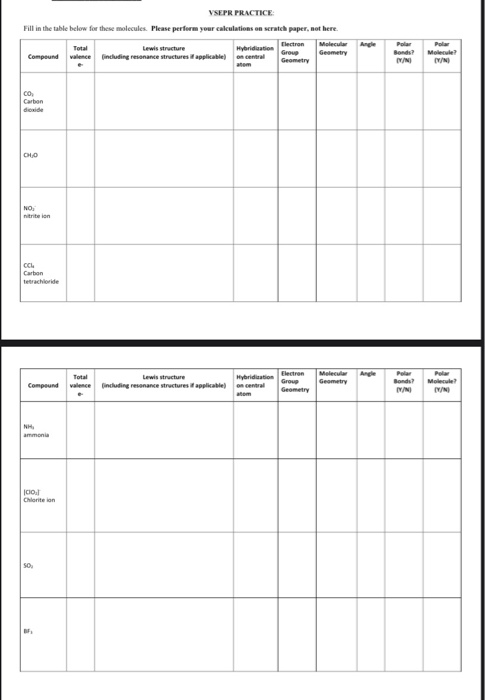VSEPR PRACTICE Fill in the table below for these molecules. Please perform your calculations on scratch paper, not here. Lewis structure Hybridisation lectron Molecular Group Compound Geometry valence including resonance structures is applicable) en central Geometry Angle Bonds? IN Polar Molecule? co Carbon dioxide NO nitrition CCM Carbon Tetrachloride Total Lewis tructure (including resonance structures it applicable) Compound Hybridisation o central Electron Group Geometry Molecular Geometry Bonds? Molecule? NH ammonia Col Chloritelon SO Angle Total Lewis structure Hybridization valence (including...

• ### Lewis Dot Structures describe the connectivity of the atoms in a species. a) Determine the centra...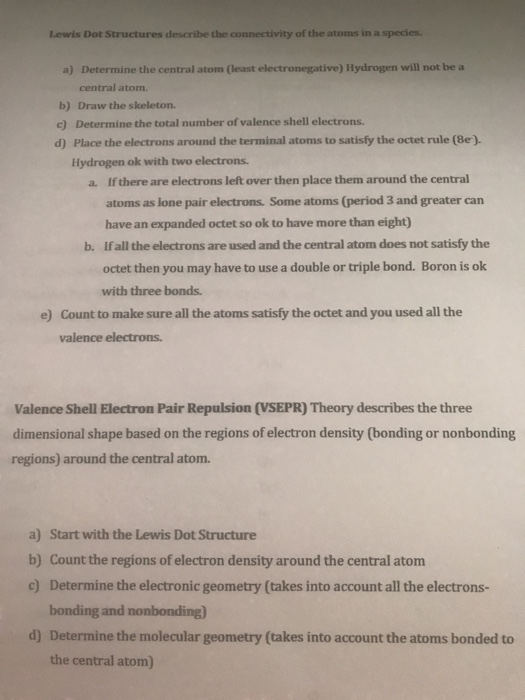Lewis Dot Structures describe the connectivity of the atoms in a species. a) Determine the central atom (least electronegative) Hydrogen will not be a central atom. b) Draw the skeleton. c) Determine the total number of valence shell electrons d) Place the electrons around the terminal atoms to satisfy the octet rule (Be). Hydrogen ok with two electrons. If there are electrons left over then place them around the central atoms as lone pair electrons. Some atoms (period 3 and...

• ### iodine pentafluoride, IFs xenon tetrafluoride, XeF. #valence electrons valence electrons: Lewis structure indicat...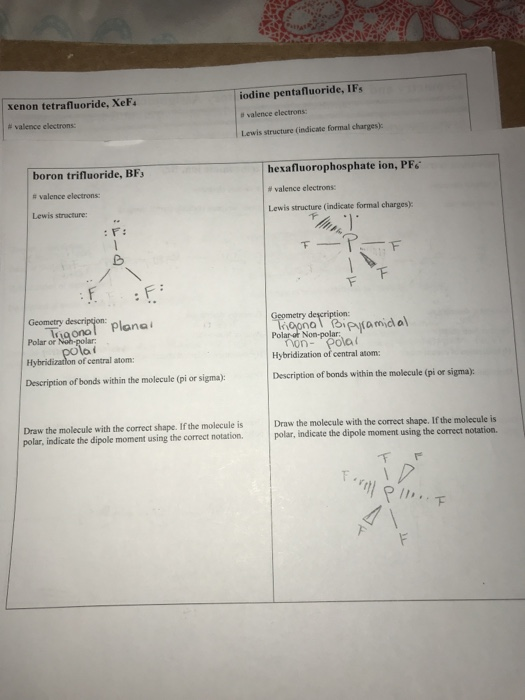iodine pentafluoride, IFs xenon tetrafluoride, XeF. #valence electrons valence electrons: Lewis structure indicate formal charges hexafluorophosphate ion, PF6 boron trifluoride, BF3 #valence electrons #valence electrons: Lewis structure indicate formal charges): Lewis structure: FTF E Geometry description: Trigona planai polai Hybridization of central atom: Geometry description: Trigonal Bipyramidal Polar of Non-polar: Ton- pola Hybridization of central atom: Polar or Non-polar: Description of bonds within the molecule (pior sigma): Description of bonds within the molecule (pi or sigma): Draw the molecule with...

• ### Covalent Molecules and Lewis Structure Experiment 5 A. For each compound, show the calculation of the...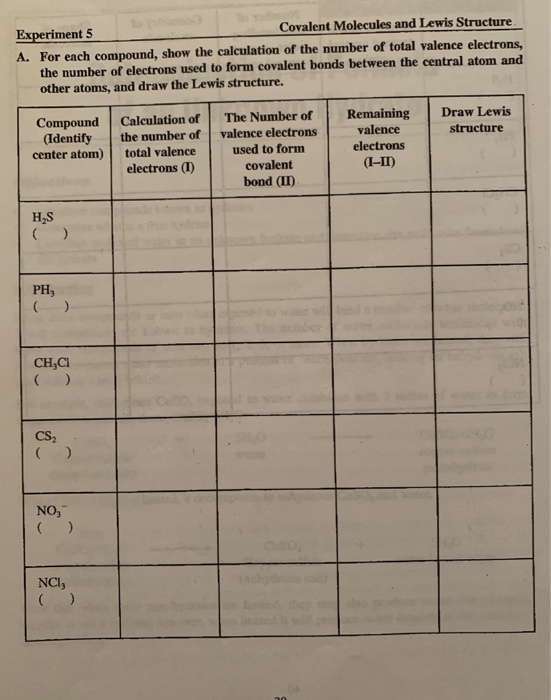Covalent Molecules and Lewis Structure Experiment 5 A. For each compound, show the calculation of the number of total valence electrons, the number of electrons used to form covalent bonds between the central atom and other atoms, and draw the Lewis structure. Remaining valence electrons Draw Lewis The Number of Calculation of Compound (Identify center atom) structure valence electrons used to form covalent the number of total valence electrons (I) (I-II bond (II) HS ( ) PH ( ) CH,CI...

• ### c2h2i2 Note: Compounds 13-16 below, will be provided in lab. 13. KHI Total # of Valence...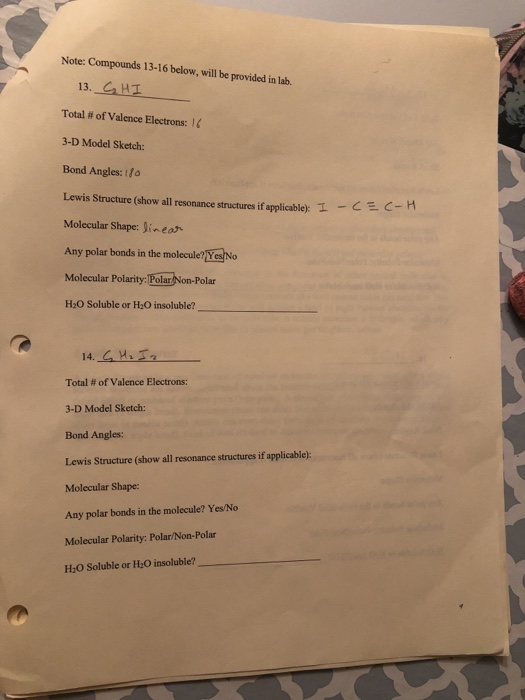c2h2i2 Note: Compounds 13-16 below, will be provided in lab. 13. KHI Total # of Valence Electrons: 16 3-D Model Sketch: Bond Angles: 10 Lewis Structure (show all resonance structures if applicable T- Molecular Shape: linear Any polar bonds in the molecule? Yes No Molecular Polarity:Polar Non-Polar H20 Soluble or H2O insoluble? 14. S Hz Ja Total # of Valence Electrons: 3-D Model Sketch: Bond Angles: Lewis Structure (show all resonance structures if applicable): Molecular Shape: Any polar bonds in...

• ### Consider the compound whose formula is shown below. C2H40 1) Calculate the total number of valence...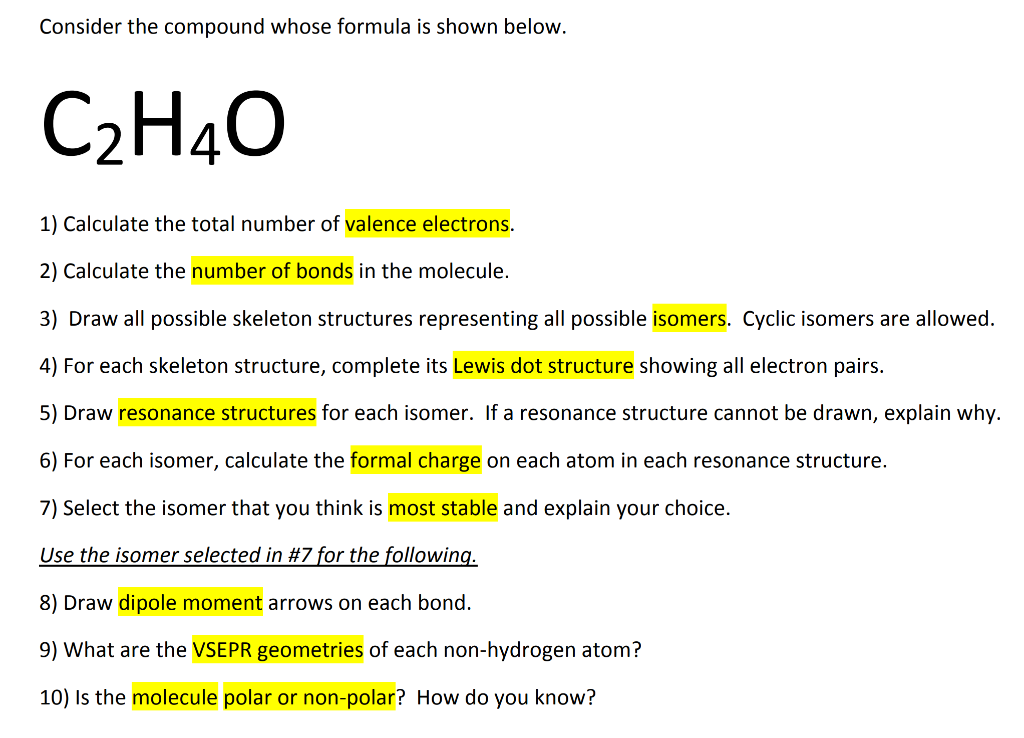Consider the compound whose formula is shown below. C2H40 1) Calculate the total number of valence electrons. 2) Calculate the number of bonds in the molecule. 3) Draw all possible skeleton structures representing all possible isomers. Cyclic isomers are allowed. 4) For each skeleton structure, complete its Lewis dot structure showing all electron pairs. 5) Draw resonance structures for each isomer. If a resonance structure cannot be drawn, explain why. 6) For each isomer, calculate the formal charge on each...

Free Homework App

Scan Your Homework
to Get Instant Free Answers
Need Online Homework Help?

Get Answers For Free
Most questions answered within 3 hours.